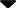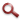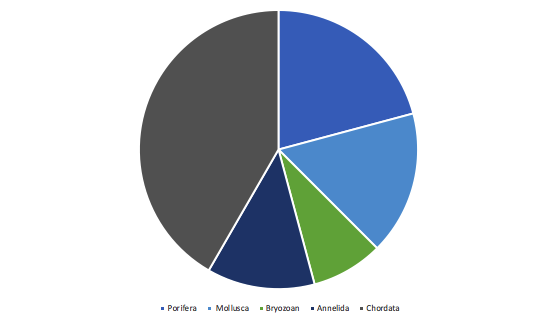Site WebYou are here:

## Student Project FiguresFigure 7 Figure 7: Chart showing the composition of the 2021 plates by phyla. Chordata = 42%, Porifera = 21%, Mollusca = 17%, Bryozoan = 8%, Annelida = 13%. Figure 3 Figure 4 Figure 5 Figure 6 Figure 7